Next: Step 2 Up: Near Optimal Multiple Sequence Previous: Tree Alignment (TA) and

#### SP alignment and Maximum Weight Trace:

The MSA and maximum weight trace methods [5,14,15,24] differ from the others because they compute an optimal alignment with respect to a well-defined MSA scoring (sum-of-pairs). This is an exact algorithm and the running time is typically O(kn) which is only practical for a very small number (n) and length (k) of sequences. In addition, the scoring function that is used does not reflect the structure of the underlying evolutionary tree, unless the scoring function is weighted, in which case a tree must be known (or constructed). A better and more detailed overview of multiple sequence alignment methods is presented in [27,19,38].
Error Bounds and Performance
Guarantees There is a bounded-error approximation method by Gusfield  for the sum of pairs (SP) measure that calculates an MSA with a score that is never more than twice that of the optimal SP score. Here a scoring method is used where lower scores correspond to better alignments. Another bound is presented by Bafna, Lawler and Pevzner . Their method aligns n strings and produces an MSA whose SP score can never exceedtimes the optimal SP score, for any chosen q. For any fixed value of q, the running time of the method is polynomial in k (the assumed string length of each string) and n. As q increases, so does the provable accuracy of the result, but the worst-case running time also increases.
In this extended abstract we present an algorithm that runs in O(n2 k2) time and produces an MSA whose score is at least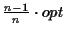. The score of the alignment represents the probability of the entire evolutionary configuration. In the following sections we briefly explain the scoring function. See  for a more detailed exposition. We then describe the algorithm for the calculation of MSAs and present experimental results. Methods Sum of Pairs versus Circular Sum measure

Definition 0.6   The tree T(S)=(V, E, L) is a binary, leaf labeled tree with leafset.

In our context a tree T(S) associated with a set of sequences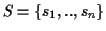is the tree that corresponds to the evolutionary history of the sequences of S. The internal nodes V represent (usually unknown) ancestor sequences. To calculate the score with the SP measure , all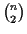scores of the pairwise alignments are added up. SP methods are obviously deficient from an evolutionary perspective. Consider a tree (Figure 2) constructed for a family containing five proteins. The score of a pairwise alignmentevaluates the probability of evolutionary events on edges (u, A) and (u, B) of the tree; that is, the edges that represent the evolutionary distance between sequence A and sequence B. Likewise, the score of a pairwise alignment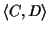evaluates the likelihood of evolutionary events on edges (C, w), (w, v) and (v, D) of the tree.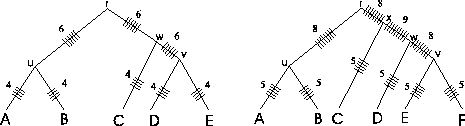By adding ticks'' to the evolutionary tree that are drawn each time an edge is evaluated when calculating the SP score (Figure 2), it is readily seen that with the SP method different edges of the evolutionary tree of the protein family are counted a different numbers of times. In the example tree on the left side that corresponds to the MSA of Figure 1, edges (r, u), (r, w) and (w, v) are each counted six times by the SP method, while edges (u, A), (u, B), (v, D), (v, E), and (w, C) are each counted four times. It gets worse as the tree grows (see tree on the right). There is no theoretical justification to suggest that some evolutionary events are more important than other ones. Thus, SP methods are intrinsically problematic from an evolutionarily perspective for scoring MSAs. This was the motivation to developed a scoring method that evaluates each edge equally. In addition, we wanted a scoring function that does not depend on the actual tree structure. Traveling Salesman

Definition 0.7   A Circular order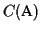of an MSA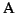is any tour through a tree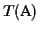where each edge is traversed exactly twice, and each leaf is visited once.

When a tree is traversed in a circular order, that is, from leaf A to B, from B to C, from C to D, from D to E and then back from E to leaf A (Figure 3), all edges are counted exactly twice, independent of the tree structure. A circular order is the shortest possible tour through a tree where each leaf is visited once (shortest means smallest sum of edge lengths)(see Figure 3) . Since we do not want to rely on any tree, the problem we consider is to find such an order without having any information about the tree structure. To solve this problem we reduce it to the symmetric Traveling Salesman Problem (TSP): given is a matrix M that contains thedistances of n cities [23,22]. The problem is to find the shortest tour where each city is visited once. We use a modified version of the problem: in our case, the cities correspond to the sequences and the distances are the scores of the pairwise alignments. In addition, we are interested in the longest, not shortest tour, as our scores represent probabilities and we are interested in the maximum probability 1.

Problem 0.2 (TSP problem)   Given is a set of sequencesand the corresponding scores of the optimal pairwise alignments. The problem is to find the longest tour where each sequence is visited once.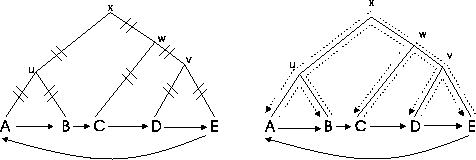Both the construction of optimal evolutionary trees [8,20] and the Traveling Salesman Problem are NP complete. But the TSP is very well studied and optimal solutions can be calculated within a few hours for up to 1000 cities and in a few seconds for up to 100 cities, whereas the construction of evolutionary trees is still a big problem. There are heuristics for large scale problems that calculate near optimal solutions that are within 1% to 2% of the optimum [29,13]. For real applications we have seldom more than 100 sequences to compare simultaneously, and the calculation of the optimal TSP solution usually takes only a small fraction of the time it takes to compute all pairwise alignments to derive the scores.

Definition 0.8   The TSP order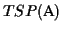of an MSAis the order of the sequences that is derived from the optimal solution of a TSP.

In summary, we can compute a circular orderwithout knowing. For a more detailed explanation see . MSA scoring function

Definition 0.9   The function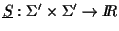is defined as follows: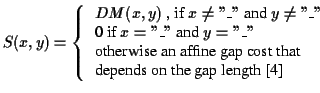DM is an entry in the Dayhoff matrix .

Our scoring function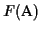of an MSA is similar to the sum-of-pairs measure, except that we do not add all scores of all pairwise alignments, but only the scores of the pairwise alignments in the circular order C divided by two. It is the sum-of-pairs in circular order:

Definition 0.10   The scoring functionof an MSAis: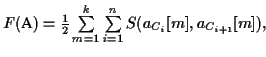with Cn+1 = C1

where k is the length of a sequence in the MSA, n is the number of sequences, C is the TSP ordering, aCi is a sequence in the MSA, and so the aCi[m] are amino acids or gap characters within that sequence. S(x, y) is the score of aligning the symbol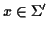with the symbol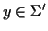. The function S(x, y) is defined above (see Definition 2.4). Circular Tours and Gaps Gaps play a special role in MSAs: the process of calculating an MSA can be viewed as inserting gaps at the correct places. Assume we are given an MSAand we know the correct tree. Insertions and deletions (indels) are evolutionary events that can be placed in the evolutionary tree (see Figure 5). The result of a deletion event are gaps that appear in all sequences of the subtree where the event took place. In contrast, when the event was an insertion, gaps appear in all sequences that are not in the subtree below the event.

Definition 0.11   A gap block B is a set of gaps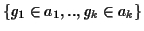where the gaps have the following properties: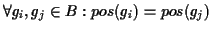and len(gi) = len(gj).

Example: In the alignment of Figure 4, there are 4 indel events and therefore 4 gap blocks. The first gap block consists of two gaps that appear in sequences A and B. We assume we have the correct tree and we also assume that we know the place of the root. In this case the event must be a deletion in the ancestor of sequences A and B. Accordingly, event 2 is an insertion in sequence D, event 3 represents a deletion in the ancestor of D and E, and finally event 4 is an insertion in sequence C.

Figure 4: An MSA and a table containing the pairwise scores


Event     1         2        3         4
A: RPCVCP___VLRQAAQ__QVLQRQIIQGPQQLRRLF_AA
B: RPCACP___VLRQVVQ__QALQRQIIQGPQQLRRLF_AA
C: KPCLCPKQAAVKQAAH__QQLYQGQLQGPKQVRRAFRLL
D: KPCVCPRQLVLRQAAHLAQQLYQGQ____RQVRRAF_VA
E: KPCVCPRQLVLRQAAH__QQLYQGQ____RQVRRLF_AA


 A B C D E A 0 336 27 44 110 B 336 0 79 56 99 C 27 79 0 171 176 D 44 56 171 0 327 E 110 99 176 327 0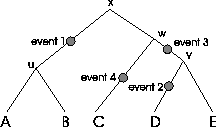The table to the right shows the pairwise scores of the sequences. It is easy to verify that the shortest tour is (A, B, C, D, E, A) and that it is a circular tour in the corresponding tree (see Figure 1). The score of the MSA is the sum of pairwise alignments in circular order divided by two, so. Here the MSA has the optimal score. Note that the sequences above are ordered in a circular way. Whenever sequences are in a circular order with respect to the evolutionary tree, the gaps that belong to the same evolutionary event appear in a gap block (ignoring the breaking of the circularity). Results The fact that gaps originating from the same event form gap blocks if a circular tour is known simplifies the task of calculating an MSA.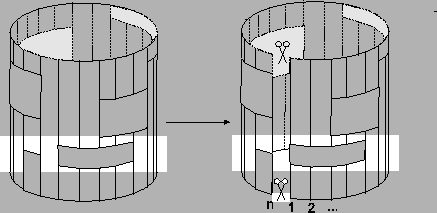In Figure 6, a schematic representation of an MSA is shown, where the vertical lines are sequences in the alignment. The rectangles represent gap blocks, and the cylinder itself represents the circular order of the tree. Once a circular orderis known, we have to select a starting point in the cycle. In principle it does not matter where we start, but as we will see later it is simpler if we cut the cycle in a place where the sequences are farther apart. This is the two sequences in the cycle with the smallest similarity score. We then renumber the sequences with respect to the cycle starting from that point (see Figure 6). Algorithm

Definition 0.12   Similar to the definition of the score of an MSA we define the partial score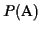of an MSA: it is the sum-of-pairs in circular order except for the last term: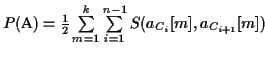(see 1.3).

The partial score of the MSA in Figure 4 is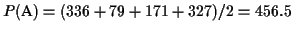.
Assume we are given n sequences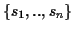which are ordered with respect to a circular order C. Assume further that the first k sequences are already aligned and build an MSAwith optimal partial score (see Figure 7). We refer to the unaligned input sequences with si, to the sequences in the MSA with ai and to the sequences in an optimal pairwise alignment with ti.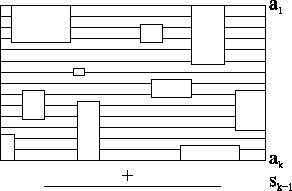The algorithm has four steps:
1.
take sequence sk and calculate an optimal pairwise alignment with the next sequence sk+1. The sequences in this alignment with the gaps are called tk and tk+1 (see Figure 8).
2.
insert all gaps from tk that are not already present in ak into all previously aligned sequences(see Figure 9).
3.
take all the gaps that were present in ak before step 2), and insert them in both, tk and tk+1, except for the gaps that are already present in tk (see Figure 9).
4.
at this point ak = tk and ak+1 = tk+1. We add ak+1 to the alignment and increment k by one (see Figure 9).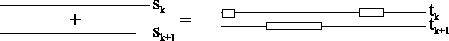We now discuss steps 2 to 4 and show that in each step the MSA has an optimal partial score:Next: Step 2 Up: Near Optimal Multiple Sequence Previous: Tree Alignment (TA) and
Chantal Korostensky
1999-07-14